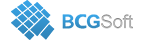BCGSuite for MFC

This enumeration describes a data label content.

Enumerator
LC_DEFAULT_CONTENT

The default content built from category name and all ternary values percentages.

LC_SERIES_NAME

Series name.

LC_CATEGORY_NAME

Category name of specified data point.

LC_VALUE

Y value.

LC_PERCENTAGE

Percentage (for Pie, Pyramid, Funnel)

LC_X_VALUE

X value (which can be either a category name or X value for scattered charts)

LC_BUBBLE_SIZE

Bubble size for Bubble series.

LC_Y1_VALUE

Y1 value.

LC_Y2_VALUE

Y2 value.

LC_A_TERNARY_PERCENTAGE

Percentage of component A (Y)

LC_B_TERNARY_PERCENTAGE

Percentage of component B (Y1)

LC_C_TERNARY_PERCENTAGE

Percentage of component C (Y2)

LC_DP_INDEX

Data point index in the series.

LC_A_TERNARY_VALUE

Value of component A (Y)

LC_B_TERNARY_VALUE

Value of component B (Y1)

LC_C_TERNARY_VALUE

Value of component C (Y2)

LC_BUBBLE_VALUES

All commonly used bubble values: Y value, X value and bubble size.

LC_ALL_BUBBLE_VALUES

All bubble values: series name, Y value, X value, bubble value.

LC_VALUE_PERCENTAGE

Value and percentage (for Pie, Pyramid, Funnel)

LC_SERIES_VALUES

Commonly used values: series name, Y value, X value.

LC_SERIES_CATEGORY_VALUE

Commonly used values: series name, category name, Y value.

LC_PIE_NAME_PERCENTAGE

Commonly used Pie, Pyramid and Funnel values: category name and percentage.

LC_ALL_TERNARY_VALUES

All ternary values: A value (Y), B value (Y1), C value (Y2)

LC_ALL_TERNARY_PERCENTAGE

Commonly used ternary values: Percentage of component A (Y), Percentage of component B (Y1), Percentage of component C (Y2)